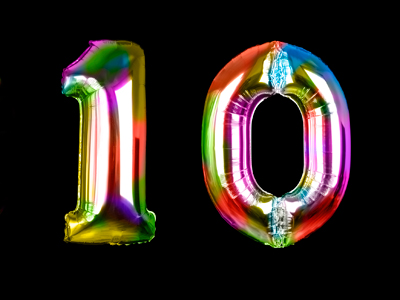In order to change 0.023 to 0.23, we multiply by 10.

# Decimal Numbers 3

This Math quiz is called 'Decimal Numbers 3' and it has been written by teachers to help you if you are studying the subject at elementary school. Playing educational quizzes is an enjoyable way to learn if you are in the 3rd, 4th or 5th grade - aged 8 to 11.

It costs only \$12.50 per month to play this quiz and over 3,500 others that help you with your school work. You can subscribe on the page at Join Us

Decimal numbers contain decimal points, on the left of which are units and on the right tenths, hundredths and thousandths. We use decimal numbers as an alternative to fractions to describe numbers that are smaller than a unit. For example, the fraction 18 could also be written as 0.125. Here we have 1 tenth, 2 hundredths and 5 thousandths. Decimal numbers make addition, subtraction, multiplication and division of fractions much easier. For this reason we have decimal measurements like meters and kilos which all use tenths, hundredths and thousandths.

1.
What is 1.23m + 63cm?
1.86m
74.23m
7.53m
186m
Convert the cm into meters and you will have 1.23 + 0.63 giving 1.86m
2.
Which is the largest of these numbers?
0.3
0.003
0.033
0.03
310 is larger than 3100, 331,000 or 31,000
3.
Which is the smallest of these numbers?
0.001
0.1
0.01
0.011
11,000 is less than 111,000, 1100 or 110
4.
How is 0.7 changed to 0.007?
Multiply by 10
Divide by 100
Divide by 10
Multiply by 100
When we divide by 100 we move the digits two places to the right
5.
What is the next number in the sequence 0.007, 0.008, 0.009?
0.001
0.1
0.01
1
The numbers are going up by 0.001, or 11,000 every time
6.
Which is the decimal fraction equivalent to nine and two thousandths?
9.002
9.2
9.02
0.092
Units are on the left of the decimal point
7.
How is 0.023 changed to 0.23?
Multiply by 10
Divide by 100
Divide by 10
Multiply by 100
When we multiply by 10 the digits move one place to the left
8.
What is the figure 8 worth in 2.089?
8 units
8 hundredths
8 tenths
8 thousandths
2.089 is 2 units, 0 tenths, 8 hundredths and 9 thousandths
9.
What does the digit 1 represent in 0.251?
1 tenth
1 thousandth
1 unit
1 hundredth
Remember - tenths, hundredths, thousandths
10.
Which is a decimal fraction halfway between 6.52 and 6.53?
6.55
6.521
6.525
6.531
There are 10 thousands in a hundredth so half of a hundredth is 5 thousandths
Author:  Amanda Swift